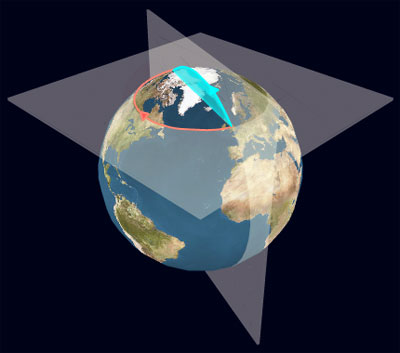#### You may also like### How Many Geometries Are There?

An account of how axioms underpin geometry and how by changing one axiom we get an entirely different geometry.### When the Angles of a Triangle Don't Add up to 180 Degrees

This article outlines the underlying axioms of spherical geometry giving a simple proof that the sum of the angles of a triangle on the surface of a unit sphere is equal to pi plus the area of the triangle.### Flight Path

Use simple trigonometry to calculate the distance along the flight path from London to Sydney.

# Over the Pole

##### Age 16 to 18 Challenge Level:Compare the distance between two points diametrically opposite each other on a line of latitude by travelling around the line of latitude in the first case and by travelling directly over the nearest pole along a line of longitude in the other case.

Make this comparison for different lines of latitude and show that the ratio of the distances tends to a limit as the lines of latitude get nearer to the pole.

The Earth Animation requires Flash Player version 9. Click on the Earth to draw the lines of latitude and longitude. Move the mouse pointer to the window edges to navigate.